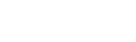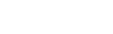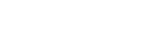# Decimals Questions and Answers - Form 1 Topical Mathematics

## Questions

1. Use a calculator to find;
1. 8754.3 x 53.84
2. 0.8341 + 8.72
3. Hence find:2. Express the recurring decimal below to a fraction 5.72 and leaving your answer in the form a/b where a and b are whole numbers
3. Evaluate:- 0.38 x 0.23 x 2.7 without using tables or a calculator
4. Without using mathematical tables or calculator, evaluate:Leaving the answer as a fraction in its simplest form.
5. Find without using a calculator, the value of :1.
1. 471331.512
2. 7.273352
3. 40.16649692
2. Let r = 5.722222………………..
10r = 57.22222……………….
100r = 572.22222…………..
100r = 572.2222……………
10r = 57.222 ………….
90r = 515
3. 38 x 23 x 27 x 100 x100000
114 x 575
= 36 For elimination of decimals For correct answer only
4. 84 x 132 x 35
287 x 560
= 99/41
5. 12 x 0.25 – 12.4 ÷ 0.4 x 3
⅛ of 2.56 + 8.68
3 – 31 x 3
0.32 + 8.68
-90/9
= -10

• ✔ To read offline at any time.
• ✔ To Print at your convenience
• ✔ Share Easily with Friends / Students